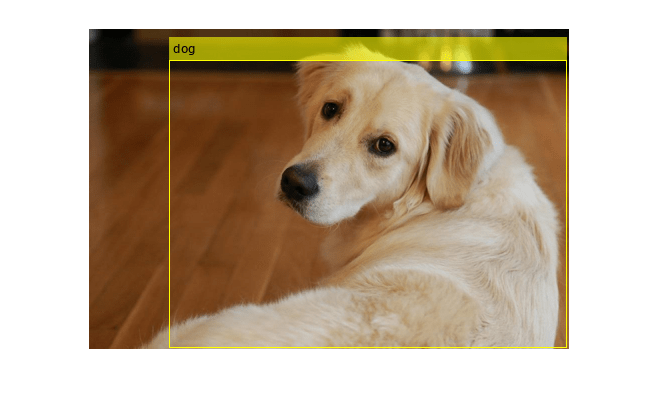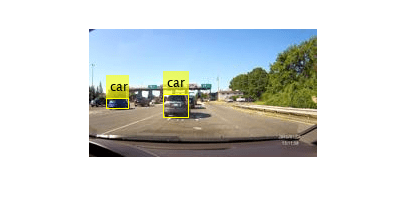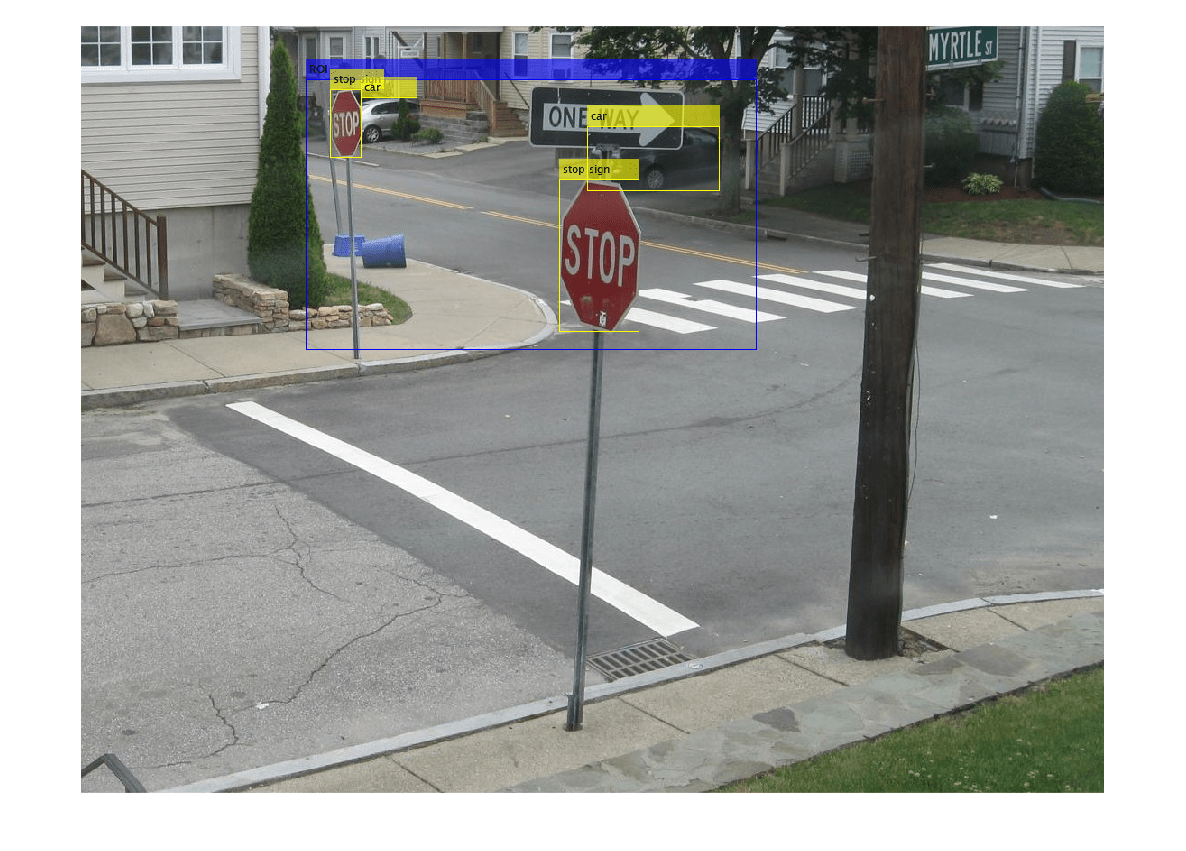# detect

Detect objects using YOLO v4 object detector

Since R2022a

## Syntax

``bboxes = detect(detector,I)``
``````[bboxes,scores] = detect(detector,I)``````
``[bboxes,scores,labels] = detect(detector,I)``
``detectionResults = detect(detector,ds)``
``[___] = detect(___,roi)``
``[___] = detect(___,Name=Value)``

## Description

example

````bboxes = detect(detector,I)` detects objects within a single image or an array of images, `I`, using a you only look once version 4 (YOLO v4) object detector, `detector`. The `detect` function automatically resizes and rescales the input image to match that of the images used for training the detector. The locations of objects detected in the input image are returned as a set of bounding boxes.```

example

``````[bboxes,scores] = detect(detector,I)``` also returns the class-specific confidence scores for each bounding box.```

example

````[bboxes,scores,labels] = detect(detector,I)` returns a categorical array of labels assigned to the bounding boxes. The labels for object classes are defined during training.```

example

````detectionResults = detect(detector,ds)` detects objects within all the images returned by the `read` function of the input datastore `ds`.```

example

````[___] = detect(___,roi)` detects objects within the rectangular search region `roi`, in addition to any combination of arguments from previous syntaxes.```
````[___] = detect(___,Name=Value)` specifies options using one or more name, value arguments. NoteTo use the pretrained YOLO v4 object detection networks trained on COCO dataset, you must install the Computer Vision Toolbox™ Model for YOLO v4 Object Detection. You can download and install the Computer Vision Toolbox Model for YOLO v4 Object Detection from Add-On Explorer. For more information about installing add-ons, see Get and Manage Add-Ons. To run this function, you will require the Deep Learning Toolbox™. ```

## Examples

collapse all

Specify the name of a pretrained YOLO v4 deep learning network.

`name = 'tiny-yolov4-coco';`

Create YOLO v4 object detector by using the pretrained YOLO v4 network.

`detector = yolov4ObjectDetector(name);`

Detect objects in an unknown image by using the pretrained YOLO v4 object detector.

```img = imread('sherlock.jpg'); img = im2single(imresize(img,0.5)); [bboxes,scores,labels] = detect(detector,img,Threshold=0.4)```
```bboxes = 1×4 single row vector 80.9433 31.6083 398.4628 288.3917 ```
```scores = single 0.4281 ```
```labels = categorical dog ```

Display the detection results.

```detectedImg = insertObjectAnnotation(img,'Rectangle',bboxes,labels); figure imshow(detectedImg)```Load a pretrained YOLO v4 object detector.

`detector = yolov4ObjectDetector("csp-darknet53-coco");`

Read the test data and store as an image datastore object.

```location = fullfile(matlabroot,'toolbox','vision','visiondata','vehicles'); imds = imageDatastore(location);```

Detect objects in the test dataset. Set the `Threshold` parameter value to 0.4 and `MiniBatchSize` parameter value to 32.

`detectionResults = detect(detector,imds,Threshold=0.4,MiniBatchSize=32);`

Read an image from the test dataset and extract the corresponding detection results.

```num = 20; I = readimage(imds,num); bboxes = detectionResults.Boxes{num}; labels = detectionResults.Labels{num}; scores = detectionResults.Scores{num};```

Perform non-maximal suppression to select strongest bounding boxes from the overlapping clusters. Set the `OverlapThreshold` parameter value to 0.5.

```[bboxes,scores,labels] = selectStrongestBboxMulticlass(bboxes,... scores,labels,OverlapThreshold=0.5);```

Display the detection results.

`results = table(bboxes,labels,scores)`
```results=2×3 table bboxes labels scores ____________________________________ ______ _______ 17.818 69.966 23.459 11.381 car 0.90267 75.206 66.011 26.134 23.541 car 0.58296 ```
```detectedImg = insertObjectAnnotation(I,"Rectangle",bboxes,labels); figure imshow(detectedImg)```Load a pretrained YOLO v4 object detector.

`detector = yolov4ObjectDetector("csp-darknet53-coco");`

`img = imread("stopsign.jpg");`

Specify a region of interest (ROI) within the test image.

`roiBox = [250 60 500 300];`

Detect objects within the specified ROI.

`[bboxes,scores,labels] = detect(detector,img,roiBox);`

Display the ROI and the detection results.

```img = insertObjectAnnotation(img,"Rectangle",roiBox,"ROI",Color="blue"); detectedImg = insertObjectAnnotation(img,"Rectangle",bboxes,labels); figure imshow(detectedImg)```## Input Arguments

collapse all

YOLO v4 object detector, specified as a `yolov4ObjectDetector` object.

Test images, specified as a numeric array of size H-by-W-byC or H-by-W-byC-by-T. Images must be real, nonsparse, grayscale or RGB image.

• H: Height

• W: Width

• C: The channel size in each image must be equal to the network's input channel size. For example, for grayscale images, C must be equal to `1`. For RGB color images, it must be equal to `3`.

• T: Number of test images in the array. The function computes the object detection results for each test image in the array.

Data Types: `uint8` | `uint16` | `int16` | `double` | `single`

Test images, specified as a `ImageDatastore` object, `CombinedDatastore` object, or `TransformedDatastore` object containing full filenames of the test images. The images in the datastore must be grayscale, or RGB images.

Search region of interest, specified as an [x y width height] vector. The vector specifies the upper left corner and size of a region in pixels.

### Name-Value Arguments

Specify optional pairs of arguments as `Name1=Value1,...,NameN=ValueN`, where `Name` is the argument name and `Value` is the corresponding value. Name-value arguments must appear after other arguments, but the order of the pairs does not matter.

Before R2021a, use commas to separate each name and value, and enclose `Name` in quotes.

Example: `detect(detector,I,Threshold=0.25)`

Detection threshold, specified as a scalar in the range [0, 1]. Detections that have scores less than this threshold value are removed. To reduce false positives, increase this value.

Select the strongest bounding box for each detected object, specified as `true` or `false`.

• `true` — Returns the strongest bounding box per object. The method calls the `selectStrongestBboxMulticlass` function, which uses nonmaximal suppression to eliminate overlapping bounding boxes based on their confidence scores.

By default, the `selectStrongestBboxMulticlass` function is called as follows

``` selectStrongestBboxMulticlass(bboxes,scores,... RatioType="Union",... OverlapThreshold=0.5);```

• `false` — Return all the detected bounding boxes. You can then write your own custom method to eliminate overlapping bounding boxes.

Minimum region size, specified as a vector of the form [height width]. Units are in pixels. The minimum region size defines the size of the smallest region containing the object.

By default, `MinSize` is 1-by-1.

Maximum region size, specified as a vector of the form [height width]. Units are in pixels. The maximum region size defines the size of the largest region containing the object.

By default, `MaxSize` is set to the height and width of the input image, `I`. To reduce computation time, set this value to the known maximum region size for the objects that can be detected in the input test image.

Minimum batch size, specified as a scalar value. Use the `MiniBatchSize` to process a large collection of image. Images are grouped into minibatches and processed as a batch to improve computation efficiency. Increase the minibatch size to decrease processing time. Decrease the size to use less memory.

Hardware resource on which to run the detector, specified as the comma-separated pair consisting of `'ExecutionEnvironment'` and `'auto'`, `'gpu'`, or `'cpu'`.

• `'auto'` — Use a GPU if it is available. Otherwise, use the CPU.

• `'gpu'` — Use the GPU. To use a GPU, you must have Parallel Computing Toolbox™ and a CUDA®-enabled NVIDIA® GPU. If a suitable GPU is not available, the function returns an error. For information about the supported compute capabilities, see GPU Computing Requirements (Parallel Computing Toolbox).

• `'cpu'` — Use the CPU.

Performance optimization, specified as the comma-separated pair consisting of `'Acceleration'` and one of the following:

• `'auto'` — Automatically apply a number of optimizations suitable for the input network and hardware resource.

• `'mex'` — Compile and execute a MEX function. This option is available when using a GPU only. Using a GPU requires Parallel Computing Toolbox and a CUDA enabled NVIDIA GPU. If Parallel Computing Toolbox or a suitable GPU is not available, then the function returns an error. For information about the supported compute capabilities, see GPU Computing Requirements (Parallel Computing Toolbox).

• `'none'` — Disable all acceleration.

The default option is `'auto'`. If `'auto'` is specified, MATLAB® applies a number of compatible optimizations. If you use the `'auto'` option, MATLAB does not ever generate a MEX function.

Using the `'Acceleration'` options `'auto'` and `'mex'` can offer performance benefits, but at the expense of an increased initial run time. Subsequent calls with compatible parameters are faster. Use performance optimization when you plan to call the function multiple times using new input data.

The `'mex'` option generates and executes a MEX function based on the network and parameters used in the function call. You can have several MEX functions associated with a single network at one time. Clearing the network variable also clears any MEX functions associated with that network.

The `'mex'` option is only available for input data specified as a numeric array, cell array of numeric arrays, table, or image datastore. No other types of datastore support the `'mex'` option.

The `'mex'` option is only available when you are using a GPU. You must also have a C/C++ compiler installed. For setup instructions, see MEX Setup (GPU Coder).

`'mex'` acceleration does not support all layers. For a list of supported layers, see Supported Layers (GPU Coder).

## Output Arguments

collapse all

Location of objects detected within the input image or images, returned as a

• M-by-4 matrix if the input is a single test image.

• T-by-1 cell array if the input is an array of test images. T is the number of test images in the array. Each cell in the array contains a M-by-4 matrix specifying the bounding box detections.

. M is the number of bounding boxes in an image.

Each row in the matrix is a four-element vector of the form [x y width height]. This vector specifies the upper left corner and size of that corresponding bounding box in pixels.

Detection confidence scores for each bounding box, returned as a

• M- element row vector if the input is a single test image.

• T-by-1 cell array if the input is an array of test images. T is the number of test images in the array. Each cell in the array contains a M-element row vector indicating the detection scores for the corresponding bounding box.

M is the number of bounding boxes detected in an image. A higher score indicates higher confidence in the detection.

Labels for bounding boxes, returned as a

• M-by-1 categorical array if the input is a single test image.

• T-by-1 cell array if the input is an array of test images. T is the number of test images in the array. Each cell in the array contains a M-by-1 categorical vector containing the names of the object classes.

M is the number of bounding boxes detected in an image.

Detection results, returned as a 3-column table with variable names, Boxes, Scores, and Labels. The Boxes column contains M-by-4 matrices, of M bounding boxes for the objects found in the image. Each row contains a bounding box as a 4-element vector in the format [x,y,width,height]. The format specifies the upper-left corner location and size in pixels of the bounding box in the corresponding image.

## Version History

Introduced in R2022a